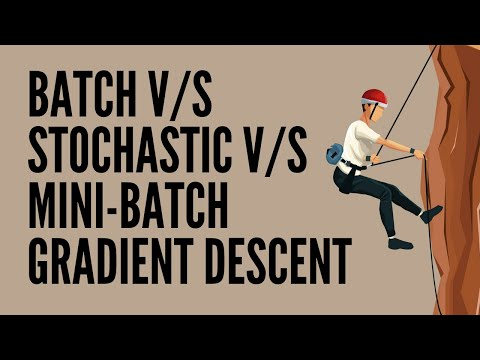# Stochastic vs Batch vs Mini-Batch Gradient Descent

Batch gradient descent computes the gradient using the whole dataset whereas Stochastic uses one training example and Mini-Batch uses a batch of 32 or 64 samples. In this video, I’ll bring out the differences of all 3 using Python.

Batch is great for convex, or relatively smooth error manifolds. In this case, we move somewhat directly towards an optimum solution, either local or global.

Stochastic gradient descent (SGD) computes the gradient using a single sample. Here, the term “stochastic” comes from the fact that the gradient based on a single training sample is a “stochastic approximation” of the “true” cost gradient. Due to its stochastic nature, the path towards the global cost minimum is not “direct” as in GD, but may go “zig-zag” if we are visualizing the cost surface in a 2D space. However, it has been shown that SGD almost surely converges to the global cost minimum if the cost function is convex.

Mini-Batch Gradient Descent combines the best of both to converge faster with less computational overhead.

In this video, I’ll walk you through all 3 variants of Gradient Descent so that the concept is clear.

## To view the video